# Harmonics

## Krystal Scale

In the Spirals of Harmony material an octave spiral was used to align sound frequencies with the Krystal Spiral expansion pattern in terms of powers of 2.  The results are summarise in the diagram over.  The key alignment is for the C note at 256Hz on the 12 o’clock line.  This in turn aligns the A note at 432Hz on the 9 o’clock line.  The other notes in the music scale align with the indicated numbers for the other clock positions, as shown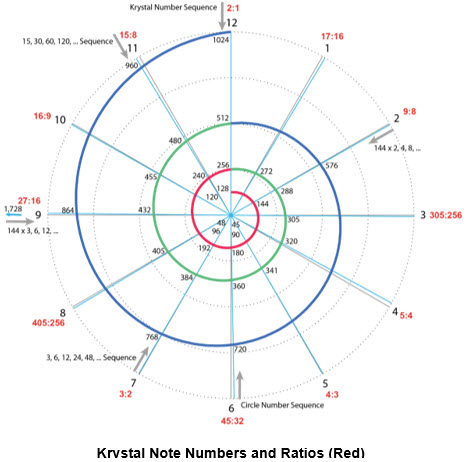We can follow this number pattern inwards towards the core of the spiral diagram to see the inner number structure as it emerges from the origin.  This generates the number distribution pattern indicated in the second diagram below.  0 representing the origin or Source lies at the center of the diagram.  We can follow the Krystal Spiral progression in terms of powers of 2, i.e. 1, 2, 4, 8, 16, 32, 64, etc., out from the origin or center along the 12 o’clock line.  The number 3 and multiples by 2, i.e. 6, 12, 24, etc., lie neatly on the 7 o’clock line.  The number 5 and multiples by 2, i.e. 10, 20, 40, etc., lie reasonably close to the 4 o’clock line.  The number 9 and multiples by 2, i.e. 18, 36, 72, etc., lie close to the 2 o’clock line, while the number 15 and multiples by 2, i.e. 30, 60, 120, etc., lie reasonably close to the 11 o’clock line.  Other points of interest are that 45, 90, 180, 360, etc. lie close to the 6 o’clock line and 27, 54, etc. lie close to the 9 o’clock line.  Intervening numbers lie in intermediate positions as shown.

This generates a pattern where most key numbers align with clock line positions.  In particular the Krystal Spiral expansion number sequence aligns with the key 12 o’clock vertical line.  This number pattern expands outwards to align with the frequency numbers in the first diagram above.  There is a high degree of Source (origin) connection and alignment in this pattern.  The numbers flow out from the origin in a coherent way

This particular number arrangement isn’t an idle curiosity nor just an interesting number pattern.  It is in fact a map of the harmonic sequence.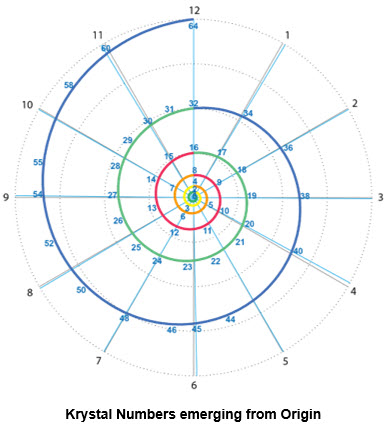## Harmonics

Musical notes carry a characteristic or fundamental frequency.  In practice this frequency rarely exists in isolation but is accompanied by multiples of that frequency.  These multiples of fundamental frequency are known as harmonics.  If we pluck a guitar string or blow on a trumpet, etc. we will get a fundamental frequency accompanied by a family of harmonics.  For example we will also get double the fundamental frequency, triple the frequency, etc. with diminishing strengths as the frequency increases.  The presence of these harmonics and their relative strengths contribute to the characteristic tone for the same note sounded on different instruments.  They help define the timbre of the note.  For example the note middle C played on a piano sounds different from that played on a violin or on organ or even when sung.  Other factors also contribute to the characteristic timbre or richness of an instrument, but the harmonics are a significant factor.

So in practice musical notes are accompanied by a family of harmonics, like the family of planets that accompany a sun.  We can see how these harmonics are distributed from the number pattern above.  The fundamental frequency corresponds to 1 in the diagram.  The second harmonic corresponds to double this frequency or 2, i.e. one loop of the spiral out along the same clock line.  The harmonics for other powers of 2, i.e. 4, 8, etc. times the fundamental frequency, correspond to still further loops out along the same clock line.  The triple-n harmonics, 3, 6, 9, etc. correspond to the positions of these number in the pattern above relative to the 1 position.

So we can use this number pattern to map the harmonic frequencies for any note.  We can rotate and expand the harmonic pattern so that the 1 lies over the location of any note in the spiral diagram.  For example we can take the 432Hz note at the 9 o’clock position in the first diagram above.  We can rotate and expand the harmonic pattern so that its 1 lies over this 432Hz position.  Then the other numbers in the harmonic pattern, 2, 3, 4, 5, etc. read off the positions of the corresponding 2nd, 3rd, 4th, 5th, etc. harmonics in the frequency chart.  This is the sense in which the number pattern in the second diagram is a map of harmonic sequence.

Given the importance of harmonics in sound expression it is important that the core harmonic sequence is aligned with the natural number sequence of the clock diagram.

What happens to this core inner number pattern when we adopt 440Hz tuning, i.e. tune middle A to 440 instead of 432Hz?  In this case the number 55 aligns with the 9 o’clock position as shown in the third diagram above.  All other number positions are rotated accordingly.  Here we see that the 1, 2, 4, 8, 16, etc. sequence no longer aligns with the 12 o’clock position, but lies between the 11 & 12 lines.  The 3, 6, 12, etc. sequence is off the 7 o’clock line between lines 6 & 7.  Likewise the 5, 10, etc. and the 15, 30, etc. sequences are off line.  Other important numbers like 45, 90, 360, etc. and 27 and others are off line.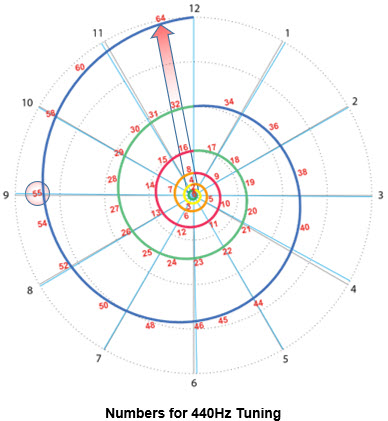All the key numbers are off line.  The only numbers that align with clock positions are either insignificant or are questionable like 55.  We have lost the core alignment with the natural stages of the unfolding spiral.  We still have harmonics for the frequencies on this scale.  But the resonance alignment with natural structures is lost.  This illustrates the importance of the Krystal Scale as a more natural frequency progression.

## Structure

The number pattern above can be used to illustrate a number of other features of the structure.  On each clock or ray line we can see the numbers expanding outwards from one spiral loop to the next in multiplication by 2.  This is illustrated by the green arrows in the diagram over.  For example on the 12 o’clock line we have 1, 2, 4, 8, 16, etc. where each number is twice the preceding one.  Likewise with all the other clock lines even though we have only illustrated a few of them.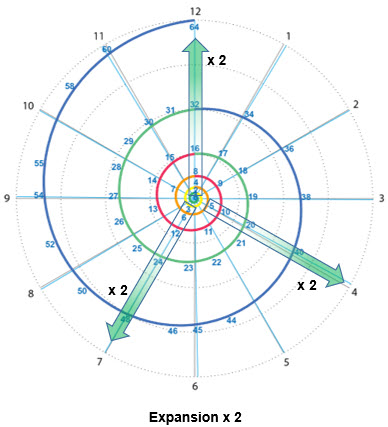This illustrates that the number 2 generates expansion out along the rays or arms of the spiral pattern.  Alternatively it can generate contraction when moving inwards.  The number 2 contributes growth and decay.

When we multiply the original number 1 by 3 we get 3 positioned on the 7 o’clock line.  This is illustrated in the following diagram where the yellow arrow shows progression from the 1 on the 12 o’clock to 3 on the 7 o’clock line.  Multiply again by 3 and we go from 3 on the 7 o’clock to 9 on the 2 o’clock line, as illustrated by the second yellow arrow.  In other words every time we multiply by 3 we rotate through 7 positions or hours on the clock diagram.  In reality we rotate through 19 positions, i.e. one full sweep around the clock and then a further 7 hours.  We can ignore the full sweep of 12 hours by dividing by 2, i.e. multiplying by 3/2 advances us by 7 hours or positions on the clock.  This 3/2 ratio has a particular significance, which will be discussed further when we look at intervals between notes.

The number 3 therefore gives us a combination of expansion and rotation.  We can eliminate some of the expansion by dividing by 2.  The 3/2 ratio effectively swaps out a 2 and replaces it with a 3.

We can continue this process in a set of stages marked by the red numbers around the outer edge of the clock diagram over.  We start from stage 0 at the original 12 o’clock position and proceed to stage 1 on the 7 o’clock line.  Then we proceed from there to stage 2 on the 2 o’clock line, then to stage 3 on the 9 o’clock line, stage 4 on the 4 o’clock line, etc.  In this way we progressively visit or mark out all 12 positions on the clock diagram.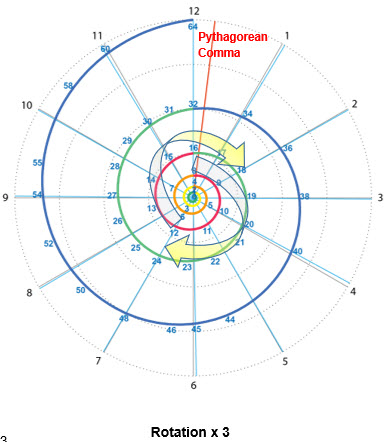The 3/2 ratio is very powerful.  It is capable of generating the 12 fold division of the octave cycles.  In fact this is how the ratios for the notes in the Pythagorean Tuning scale are developed.  They are all based on ratios between powers of 3 and 2.

After 12 stages we arrive back close to the original 12 o’clock line.  Because the 3/2 ratio doesn’t exactly match the 7 o’clock position there is a small offset.  This accumulates over 12 stages to give a more noticeable offset when we get back to 12.  So instead of arriving back exactly at 12 we reach a position further past it to the right indicated by the red line.  This offset is known as the Pythagorean Comma and is interesting in its own right.

If we apply the ratio corresponding to the Pythagorean Comma (1.0136433…) to an idealised year of 360 days we get 365 days.  This is very close to the number of days (365.25) in an actual year.  The angle corresponding to the Pythagorean Comma is 7° beyond the 12 o’clock position.  So a full cycle through 12 stages generates rotation through 367° rather than 360°.  If we apply this ratio of angles to the ideal tuning frequency of 432Hz we get 440Hz, the conventional tuning frequency.  These correspondences between offsets are interesting but may not hold major significance.

We can see therefore that the number 2 gives us expansion in the spiral of frequencies, while the number 3 additionally provides rotation.  Both are key elements of spiral behaviour.

The next highly significant number is 5.  What role does this play?  This isn’t immediately obvious.  We can swap out 3 for 5 using the ratio 5/3.  This 5/3 ratio is 12/3, which is the seed number for all the Krystic Merkaba spin speeds, i.e. all the spin speeds are multiples of this core number.  This is a highly significant ratio in terms of sacred number progressions.  It also corresponds to the 45:27 ratio, which for example is a proportion given for the Ark of the Covenant box.  This ratio brings an element of flow, openness and transcendence to the scales.  There’s a bit of work to be done to figure out the role it plays.

## Intervals

When we combine notes or tones the relationship between their frequencies is important.  The difference or ratio between the frequencies is known as the interval between them.  This is illustrated on the diagram over.

We can take the first tone on the 12 o’clock line for convenience.  We can then look at the relationship of the other tones to this.  This is illustrated by the green arrow running between the two tones.  The two notes can be played together or in sequence.  In either case the two frequencies overlap and combine for a period of time.  In this case we are starting with the C notes on the 12 o’clock line.  But we could start with any note and simply rotate the family of intervals on the diagram over to match.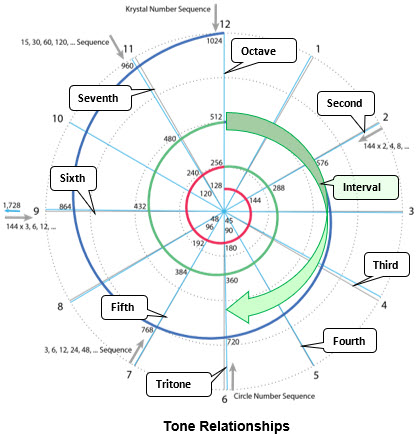The interval or frequency ratio between two tones determines how harmoniously they combine.  The simplest relationship is where two tones have the same frequency.  This is referred to as unison and simply produces the same tone again.

The most harmonious relationship arises where there is a doubling between the two frequencies.  This is referred to as the octave.  In this case both frequencies lie on the 12 o’clock line.  So where two frequencies lie on the same clock line we have an octave relationship between them.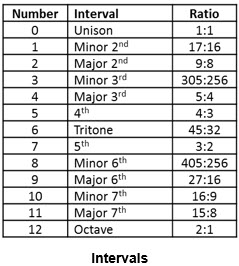This relationship can be illustrated graphically by combining a wave shape with one at double its frequency as illustrated in the graph over.  The two tones combine to produce a wave shape similar to that shown in red.  In other words if we were to set up instruments to monitor the wave forms we would get wave shapes as shown.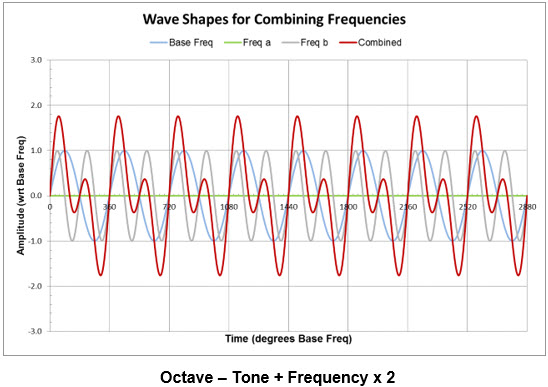It is a regular wave form with a slightly more complex structure than the original waves illustrated in the background.  The actual detail can differ depending on phasing between the tones and their relative strengths or amplitudes.  But the basic structure is as illustrated.

The next most harmonious relationship between tones arises where there is a frequency difference or ratio of 3/2.  This is referred to as the fifth interval.  It arises between two tones 7 positions or hours apart on the spiral diagram.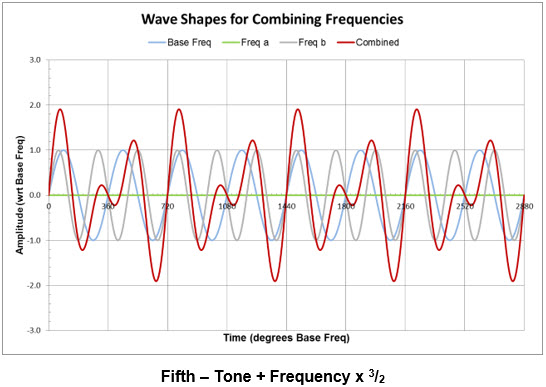The wave form associated with this interval is illustrated in the graph above.  Again it is quite regular, but with a bit more variation than for the octave case above.  While the octave stands on the power of 2 this fifth interval brings the power of 3 into play.

The regularity of the wave forms is tied in directly with the simplicity of the number ratios or intervals between the frequencies.  The simpler these number ratios the greater the regularity and the smoother the harmony between the tones.

The next simplest number ratio after 3/2 is 4/3.  This is known as the fourth interval.  It is closely related to the fifth and while not identical behaves in a somewhat similar manner.  This arises because the fourth at 5 o’clock is 5 tones or hours from the prime 12 o’clock position in the normal clockwise direction, but is 7 tones away in the counter clockwise direction for reducing frequencies.

After this we have the ratio 5/4 for the third interval.  The wave form associated with this is illustrated in the graph over.  Here again we have a regular wave shape that repeats consistently, but one with a slower undulation.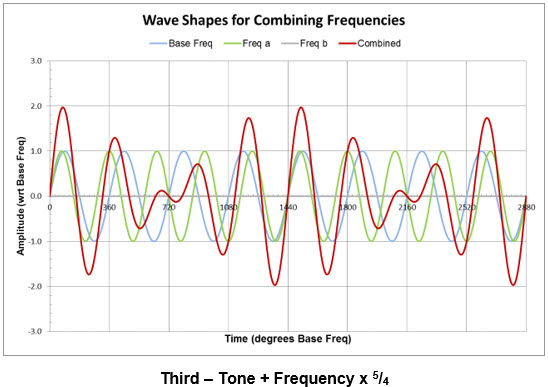Basically the lower number of the fraction determines how frequently the whole combined pattern repeats.  For 5/4 the combined pattern repeats every 4 cycles of the base or lower frequency.  We can see this in the diagram below.  A cycle of the base frequency corresponds to 360 degrees on the time scale.  4 such cycles correspond to 1440 degrees, where we see the pattern repeating.  The difference

between the upper and lower numbers in the fraction determines what happens within this cycle of repetition.  In this case it is 1 (5 – 4) so we have one cycle of the pattern within the 4 cycle repetition period.  If we had a ratio of

7/5 for example the pattern would repeat exactly every 5 cycles of the base frequency.  But within this we would have two repetitions of opposite polarity of an inner pattern.  This illustrates the significance of the numbers and the role they play in combining frequencies.   The bigger the numbers the slower the repetition of cycles and the more complex the behaviour within each cycle.

The number ratios for each of the 12 notes in an octave cycle are summarised in the ‘Intervals’ table above.  The first column shows the number of notes or scale positions between the frequencies for each interval.  The second column indicates the names associated with the intervals.  The less harmonious intervals with higher number ratios are denoted as ‘minor’ or tritone.  The other harmonious intervals are either major or singular (perfect).

The tritone interval is of particular interest.  Traditionally the 12 note scale was regarded as comprising 6 whole tones.  Each of these contained two semi-tones to make up the 12 tones.  So in these terms the note at position 6 was 3 whole tones (6 semi-tones) from the 12 o’clock position.  Hence the name tritone.  Basically it refers to a tone opposite the reference tone at 12 or to an interval where two notes are on opposite sides of the clock diagram.

The pure tritone has a relationship with the base frequency of √2 (1.414…).  This number cannot be expressed as a fraction.  It is referred to as irrational, i.e. cannot be expressed as a ratio.  This means that the wave pattern never repeats.  In this context it is regarded as the least harmonious of the intervals.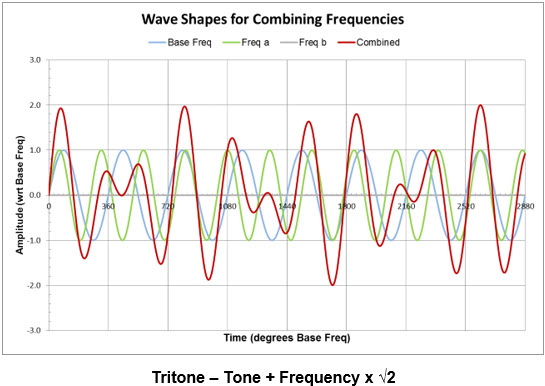The wave pattern associated with the tritone is illustrated in the graph above.  Even though there appears to be some regularity the pattern never repeats.  There is a continuous variation throughout.  When we use the 45/32 ratio for this interval we get a somewhat similar pattern.  But in this case there is a complex repetition every 32 cycles of the base frequency.

However this ratio also corresponds with the intermediate 45° rotation stages of the Kathara Grid to form the Krystal Spiral.  The 90° stages correspond to the octave positions with expansions of 2, 4, 8, etc.  The intermediate 45° stages correspond to the tritone positions on the 6 o’clock line of the octave spirals.  This is a powerful connection.  This interval also associates with the significant 45, 90, 180, 360, etc. numbers.  The tritone appears to act as a doorway in the scale.  We will look at its significance in more detail when we consider impulse and rhythm further on.

Finally a further interval of interest is the sixth in the 9 o’clock position.  The 27/16 ratio here is reasonably close to the very significant 5/3 ratio mentioned earlier.  Wherever we get notes 9 clock positions apart we are close to this 5/3 ratio.  For example in the harmonic numbers diagram near the beginning we have 27 in the 9 o’clock position and 45 nine hours further on in the 6 o’clock position.  This is a significant proportion equivalent to the 5/3 ratio and the 12/3 seed number for the Merkaba spin speeds.

## Scales

The distinction between major and minor notes and intervals creates a division within the 12 notes in an octave.  Conventionally the 7 most harmonious notes are taken to form a scale and these are the notes mostly used in a musical work.  The assumption is that they will work well together.  The most common such scale is the C major scale.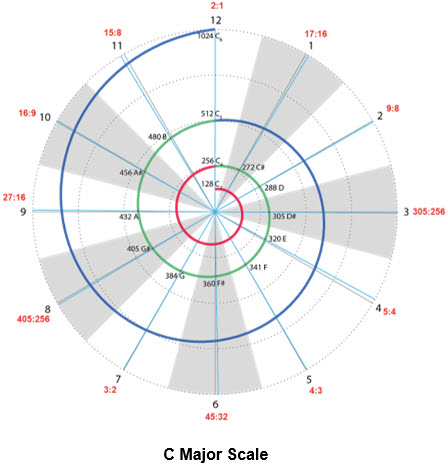The 7 notes in this scale are shown in white in the spiral diagram above.  They correspond to the major and perfect intervals in the earlier table.  They also correspond to the white keys on a piano as shown above.  The remaining 5 notes shown in grey in the spiral diagram and as black keys on a piano tend to be avoided.  The octave sets are indicated on the piano diagram below.  The blue key corresponds to middle C in the 12 o’clock position.  The yellow key likewise corresponds to middle A, the reference tuning position at 9 o’clock.

This is where we get the 7 note scales from.  In conventional note annotation these ‘white’ notes are denoted by A, starting in the 9 o’clock position, then B, C, etc. round to G in the 7 o’clock position.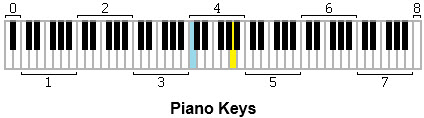These conventional note names are shown in the spiral diagram above.  The remaining ‘black’ notes then are named as sharps (#) or a step up in frequency from the preceding white note, e.g. C# for position 1.  Alternatively they can also be named as flats (♭) or a step down in frequency from the following white note, e.g. D♭ for position 1.  It’s interesting that the annotation begins at the 9 o’clock rather than the 12 o’clock position and also that this is taken as the reference point for tuning, e.g. A at 432Hz or 440Hz.  The key natural reference points are on the 12 o’clock line.

This subdivision of 12 into 7 primary and 5 secondary notes also explains the conventional numbering of the intervals in the table above.  The 7 more harmonious major and perfect intervals are numbered 1 through 7.  The other intervals are designated as minors off these or as the tritone.  The 3/2 interval arises as the fifth in this sequence hence its name as the fifth.  The reference there to 5 is somewhat accidental.  The significant relationship is with 3.

When choosing 7 note scales for particular pieces of music it is possible to rotate the pattern to start with a different frequency other than C.  Also we can start at a different point within the pattern such as the A note for an A minor scale, etc.  But the basic principles are the same.

## Beating

Where there isn’t a simple numerical relationship between frequencies we get increasingly irregular patterns of variation in their combination.  In particular where there is a small difference in frequency between tones we get a phenomenon known as ‘beating’.  An example of this is illustrated in the graph over.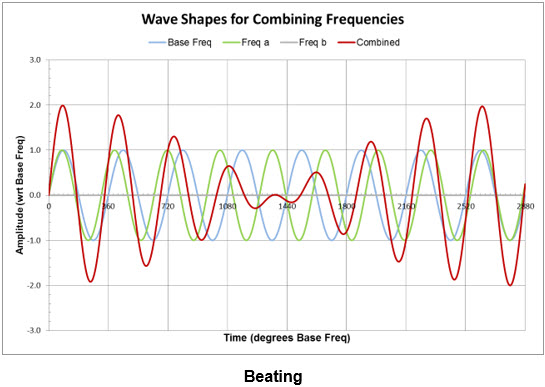In this case we get a sound pattern that increases and decreases in volume on a slow cycle.  The core frequency or carrier wave oscillates at the average of the two component frequencies.  The volume of this varies at the difference of the two frequencies involved.  So for a small difference in frequency we get a slow oscillation in volume.  This can be quite distracting or irritating.  This phenomenon is used to tune pianos for example.  Strings are tuned to eliminate beating and obtain simple tone relationships between their frequencies.

This also illustrates that when we combine frequencies we get additional components.  As we saw above we get a combined carrier frequency running at the average of the two component frequencies.  The variation in the strength or amplitude of this frequency pattern can also be considered as a frequency.  If we draw a wave pattern between the tops of the peaks and another between the bottoms or valleys to envelope the pattern we can see this second wave or frequency.  It is referred to as a modulating wave.  It has a frequency related to the difference of the component frequencies.  Generally it will be less than the component frequencies and in this context can be referred to as an undertone or sub-harmonic.

We have seen already how tones will normally carry some higher frequency components at multiples of the base frequency going up the frequency scale and known as harmonics.  There can also be sound components going down the frequency scale especially when we combine tones.  As we see from the map of the harmonic sequence above most of the harmonic components that accompany a tone enjoy a good or harmonic relationship with the base or fundamental frequency.  Likewise notes whose fundamental frequencies are harmonic will also have reasonable relationship between their respective harmonics.

When we combine tones their harmonics will also interact to create additional frequencies both going up and down the frequency scale.  This effectively establishes a cascade or stairway of frequency extending in both directions.   It indicates the power and the complexity of the issue.

## Circle of Fifths

The circle of fifths is a powerful structure based on the fifth interval (3/2).  This is illustrated in the diagram over from Wikipedia.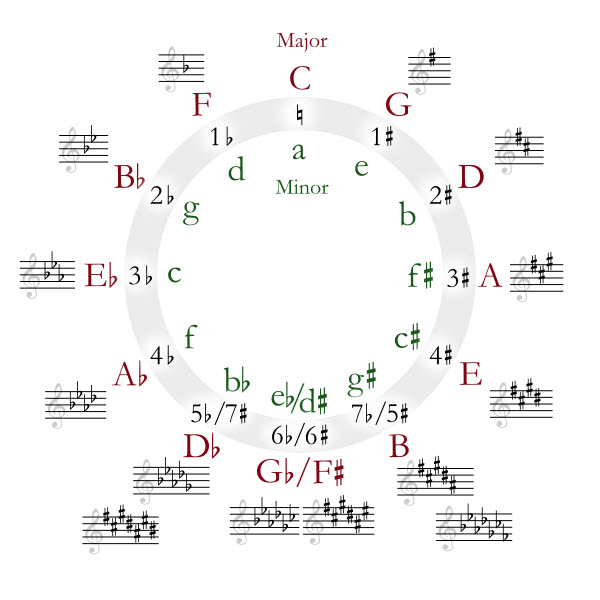## Three Transcendent Notes

Three additional notes in each octave to bring existing 12 notes up to 15.  3 extra notes correspond to dimensions 13, 14 & 15 and the primal light fields. They bridge from the 12 material dimensions through to the primal sound fields.  They enable full Source connection and could be referred to as the transcendent tones.
This combination of 15 notes in each octave then gives us a bigger field to play with.  We can now have two groups of 7 linked by the key central note.  This structure is illustrated in the number tree above.  This allows for the inclusion and utilisation of all the notes.  In other words we can build more powerful and comprehensive scales within the family of notes.

The key issue obviously is to determine the 3 extra notes in each scale.  This has still to be done.  They should be able to balance the disharmonies in the existing notes as well as opening to the next level up, i.e. the next octave.

## Impulse & Rhythm

We can superimpose the partiki phasing process on the Reuche core or on the clock pattern as indicated in the diagram over.  This indicates the relative polarities of the components – electric, magnetic, omni-polar, etc.

The interaction of the electric and magnetic polarities sets up the oscillations that underlie frequency.  All frequencies arise in this way.  This is a ‘horizontal’ process that sets up horizontal or transverse waves that travel within a dimension or frequency band.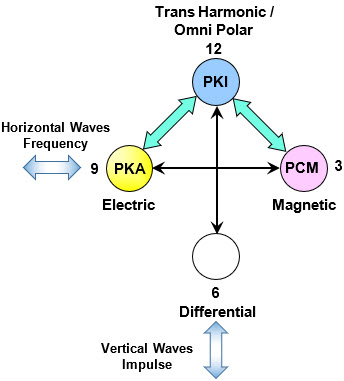There is a vertical process that moves between dimensions, bring energy, influences, etc. into and out of dimensional bands in growth and decay processes.  This process operates on the basis of longitudinal and standing waves.  It lies closer to the impulses that generate sound tones rather than the tonal frequencies they propagate.  In musical terms it is more in the nature of impulse, as in drumming, or in rhythm.  The two processes are related and work hand in hand.  The main issue is getting an appropriate balance between them.  In this context the tritone appears to be particularly significant.

## Trines

Working with sets of 3 notes allows for a balance between the 3 polarities in the Partiki Phasing process.  We have the EirA, ManA and ManU elements expressing in the Base Tone, Over Tone and Resonant Tones respectively.  This establishes a more balanced interaction between the tones.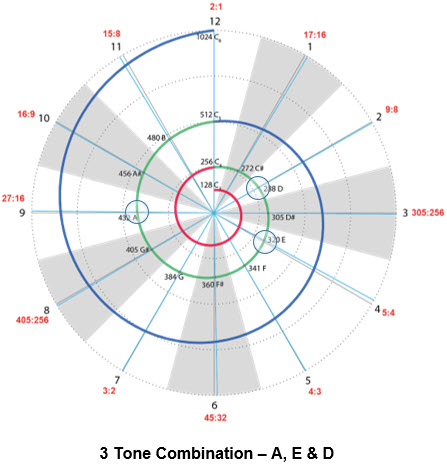We have the tools to look at the wave shapes for combinations of 3 frequencies.  An example combining the three tones A, E & D is illustrated on the chart over.  The associated wave form is also illustrated in the graph over.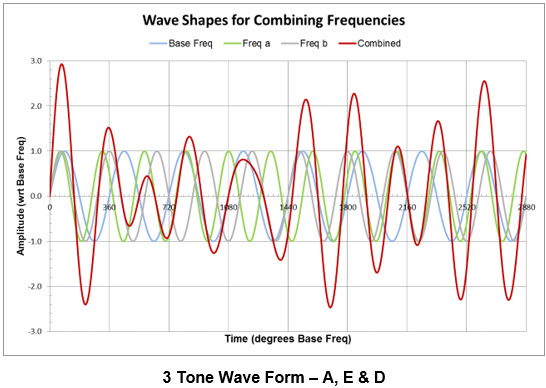Conventionally chords generally comprise 3 notes played together and structured by specific rules of organisation.  With an extended set of notes it is possible to play with more combinations, e.g. a transcendent note with one from each group of 7, etc.  This obviously involves a lot of experimentation.